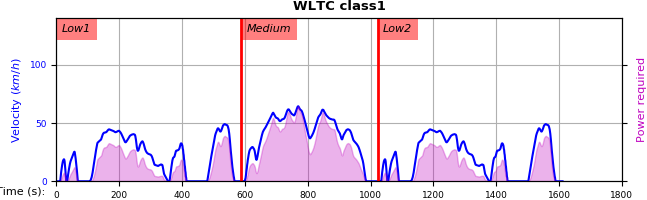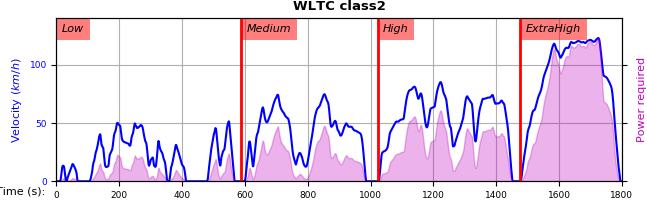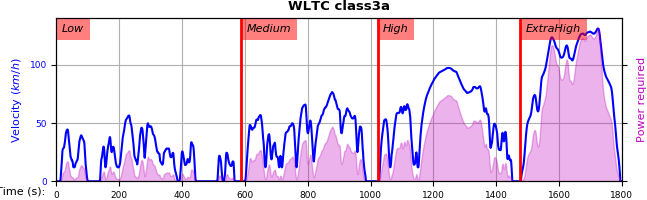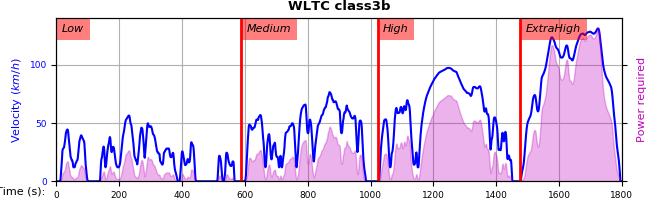# 6. Annex 2¶

:

## 6.1. Cycles¶

The WLTC-profiles for the various classes were generated from the tables of the specs above using the devtools/csvcolumns8to2.py script, but it still requires an intermediate manual step involving a spreadsheet to copy the table into ands save them as CSV.### 6.1.1. Phases¶

As reported by wltp.cycles.cycle_phases(), where phasing refers to:

• V: phases for quantities dependent on Velocity samples

• VA0: phases for Acceleration-dependent quantities starting on t=0.

• VA1: phases for Acceleration-dependent quantities starting on t=1 (e.g. Energy in Annex 7).

class

phasing

part-1

part-2

part-3

part-4

class1

V

[0, 589]

[589, 1022]

[1022, 1612]

VA0

[0, 588]

[589, 1021]

[1022, 1611]

VA1

[1, 589]

[590, 1022]

[1023, 1612]

class2

V

[0, 589]

[589, 1022]

[1022, 1477]

[1477, 1801]

VA0

[0, 588]

[589, 1021]

[1022, 1476]

[1477, 1800]

VA1

[1, 589]

[590, 1022]

[1023, 1477]

[1478, 1801]

class3a

V

[0, 589]

[589, 1022]

[1022, 1477]

[1477, 1801]

VA0

[0, 588]

[589, 1021]

[1022, 1476]

[1477, 1800]

VA1

[1, 589]

[590, 1022]

[1023, 1477]

[1478, 1801]

class3b

V

[0, 589]

[589, 1022]

[1022, 1477]

[1477, 1801]

VA0

[0, 588]

[589, 1021]

[1022, 1476]

[1477, 1800]

VA1

[1, 589]

[590, 1022]

[1023, 1477]

[1478, 1801]

### 6.1.2. Checksums¶

As computed by wltp.cycles.crc_velocity(), reported by wltp.cycles.cycle_checksums(), and identified back by wltp.cycles.identify_cycle_v_crc():

CRC32

SUM

by_phase

cumulative

by_phase

cumulative

class

part

V

A0

A1

V

A0

A1

V

V

class1

part1

9840

4438

97DB

9840

4438

97DB

11988.4

11988.4

part2

8C34

8C8D

D9E8

DCF2

90BE

4295

17162.8

29151.2

part3

9840

9840

97DB

6D1D

6D1D

F523

11988.4

41139.6

class2

part1

8591

CDD1

8A0A

8591

CDD1

8A0A

11162.2

11162.2

part2

312D

391A

64F1

A010

606E

3E77

17054.3

28216.5

part3

81CD

E29E

9560

28FB

9261

D162

24450.6

52667.1

part4

8994

8994

2181

474B

474B

F70F

28869.8

81536.9

class3a

part1

48E5

910C

477E

48E5

910C

477E

11140.3

11140.3

part2

1494

D93B

4148

403D

2487

DE5A

16995.7

28136.0

part3

8B3B

9887

9F96

D770

3F67

2EE9

25646.0

53782.0

part4

F962

F962

5177

9BCE

9BCE

2B8A

29714.9

83496.9

class3b

part1

48E5

910C

477E

48E5

910C

477E

11140.3

11140.3

part2

AF1D

E501

FAC1

FBB4

18BD

65D3

17121.2

28261.5

part3

15F6

A779

15B8

43BC

B997

BA25

25782.2

54043.7

part4

F962

F962

5177

639B

639B

D3DF

29714.9

83758.6

## 6.2. Gear selection and shift point determination for vehicles equipped with manual transmissions¶

Attention

The document below is very outdated (late 2014)!

It was an attempt to transcribe the GTR in sphinx, in order to track changes in git.

### 6.2.1. General approach¶

1. The shifting procedures described in this Annex shall apply to vehicles equipped with manual and automatic shift transmissions.

2. The prescribed gears and shifting points are based on the balance between the power required to overcome driving resistance and acceleration, and the power provided by the engine in all possible gears at a specific cycle phase.

3. The calculation to determine the gears to use shall be based on engine speeds and full load power curves versus speed.

4. For vehicles equipped with a two-range transmission (low and high), only the range designed for normal on-road operation shall be considered for gear use determination.

5. This annex shall not apply to vehicles tested according to Annex 8.

### 6.2.2. Required data¶

The following data is required to calculate the gears to be used when driving the cycle on a chassis dynamometer:

1. $$P_{rated}$$, the maximum rated engine power as declared by the manufacturer;

2. $$s$$, the rated engine speed at which an engine develops its maximum power. If the maximum power is developed over an engine speed range, $$s$$ is determined by the minimum of this range;

3. $$n_{idle}$$, idling speed;

4. $$ng_{max}$$, the number of forward gears;

5. $$n_{min\_drive}$$, minimum engine speed when the vehicle is in motion.

• for $$n_{gear} = 1$$,

(1)$n_{min\_drive} = n_{idle}$
• for $$n_{gear} = 2$$,

(2)$n_{min\_drive} = 0.9 \times n_{idle}$
• for $$n_{gear} > 2$$, $$n_{min\_drive}$$ is determined by:

(3)$n_{min\_drive} = n_{idle} + 0.125 \times (s - n_{idle})$

Higher values may be used if requested by the manufacturer;

6. $$ndv_i$$, the ratio obtained by dividing $$n$$ in $$min^{-1}$$ by $$v$$ in $$km/h$$ for each gear $$i$$, $$i=1 \text{ to } ng_{max}$$;

7. $$TM$$, test mass of the vehicle in $$kg$$;

8. $$f_0, f_1, f_2$$, driving resistance coefficients as defined in Annex 4 in $$N$$, $$N/(km/h)$$, and $$N/(km/h)^2$$ respectively;

9. $$ng_{vmax}$$, the gear in which the maximum vehicle speed is reached, and is determined as follows:

(4)$ng_{vmax} = ng_{max}\ if\ v_{max}(ng_{max}) \ge v_{max}(ng_{max}-1),$

otherwise:

(5)$ng_{vmax} = ng_{max} -1$

where:

• $$v_{max}(ng_{max})$$ is the vehicle speed at which the required road load power equals the available power $$P_{wot}$$ in gear $$ng_{max}$$;

• $$v_{max}(ng_{max}-1)$$ is the vehicle speed at which the required road load power equals the available power $$P_{wot}$$ in the next lower gear.

(6)$P_{required} = \frac{f_0 \times v_j + f_1 \times v_j^2 + f_2 \times v_j^3}{3600}$

where:

• $$v_j$$ is the vehicle speed at second j of the cycle trace, $$km/h$$.

10. $$n_{max\_95}$$, is the minimum engine speed where 95 per cent of rated power is reached;

11. (7)$n_{max}(ng_{vmax}) = ndv(ng_{vmax}) \times v_{max,cycle}$

where:

• $$v_{max,cycle}$$ is the maximum speed of the vehicle speed trace according to Annex 1;

12. $$P_{wot}(n)$$ is the full load power curve over the engine speed range from idling speed to $$n_{max}$$.

13. $$P_{wot}(n)$$ is the full load power curve over the engine speed range from idling speed to $$n_{max}$$.

## 6.3. Calculations of required power, engine speeds, available power, and possible gear to be used¶

### 6.3.1. Calculation of required power¶

For every second $$j$$ of the cycle trace, the power required to overcome driving resistance and to accelerate shall be calculated using the following equation:

(8)$P_{prequired, j} = \frac{f_0 \times v_j + f_1 \times v_j^2 + f_2 \times v_j^3}{3600} + \frac{kr \times a_j \times v_j \times TM}{3600}$

where:

• $$f0$$ is the road load coefficient, $$N$$;

• $$f1$$ is the road load parameter dependent on velocity, $$N/(km/h)$$;

• $$f2$$ is the road load parameter based on the square of velocity, $$N/(km/h)^2$$;

• $$P_{required,j}$$ is the required power at second $$j$$, $$kW$$;

• $$v_j$$ is the vehicle speed at second $$j$$, $$km/h$$;

• $$a_j$$ is the vehicle acceleration at second $$j$$, $$m/s$$,

(9)$a_j = \frac{v_{j+1} - v_j}{3.6 \times (t_{j+1} - t_j)};$
• $$TM$$ is the vehicle test mass, $$kg$$;

• $$kr$$ is a factor taking the inertial resistances of the drivetrain during acceleration into account and is set to $$1.1$$.

### 6.3.2. Determination of engine speeds¶

For any $$v_j \le 1\ km/h$$, it shall be assumed that the vehicle is standing still and the engine speed shall be set to $$n_{idle}$$. The gear lever shall be placed in neutral with the clutch engaged except one second before beginning an acceleration phase from standstill where first gear shall be selected with the clutch disengaged.

For each $$v_j \ge 1\ km/h$$ of the cycle trace and each gear $$i, i=1 \text{ to } ng_{max}$$, the engine speed $$n_{i,j}$$ shall be calculated using the following equation:

(10)$n_{i,j} = ndv_i \times v_j$

### 6.3.3. Selection of possible gears with respect to engine speed¶

The following gears may be selected for driving the speed trace at $$v_j$$:

1. all gears $$i < ng_{vmax}$$ where $$n_{min\_drive} \le n_{i,j} \le n_{max\_95}$$, and

2. all gears $$i \ge ng_{vmax}$$ where $$n_{min\_drive} \le n_{i,j} \le n_{max}(ng_{vmax})$$

If $$aj \le 0$$ and $$n_{i,j}$$ drops below $$n_{idle}$$, $$n_{i,j}$$ shall be set to $$n_{idle}$$ and the clutch shall be disengaged.

If $$aj > 0$$ and $$n_{i,j}$$ drops below $$(1.15 \times n_{idle})$$, $$n_{i,j}$$ shall be set to $$(1.15 \times n_{idle})$$ and the clutch shall be disengaged.

### 6.3.4. Calculation of available power¶

The available power for each possible gear $$i$$ and each vehicle speed value of the cycle trace $$v_j$$ shall be calculated using the following equation:

(11)$p_{available\_i,j} = P_{wot}(n_{i,j}) \times (1-(SM + ASM))$

where:

• $$P_{rated}$$ is the rated power, $$kW$$;

• $$P_{wot}$$ is the power available at $$n_{i,j}$$ at full load condition from the full load power curve;

• $$SM$$ is a safety margin accounting for the difference between the stationary full load condition power curve and the power available during transition conditions. $$SM$$ is set to $$10$$ per cent;

• $$ASM$$ is an additional exponential power safety margin, which may be applied at the request of the manufacturer. $$ASM$$ is fully effective between $$n_{idle}$$ and $$n_{start}$$, and exponentially approaching $$0$$ at $$n_{end}$$ as described by the following requirements:

If $$n \le n_{start}$$, then

(12)$ASM = ASM_0$

If $$n > n_{start}$$, then

(13)$ASM = ASM_0 \times exp(ln(\frac{0.005}{ASM_0}) \times \frac {n_{start} - n}{n_{start} - n_{end}})$

$$ASM_0, n_{start}$$ and $$n_{end}$$ shall be defined by the manufacturer but must fulfill the following conditions:

\begin{align}\begin{aligned}n_{start} \ge n_{idle},\\n_{end} > n_{start}\end{aligned}\end{align}

### 6.3.5. Determination of possible gears to be used¶

The possible gears to be used shall be determined by the following conditions:

1. The conditions of paragraph 3.3. are fulfilled, and

2. $$P_{available\_i,j} \ge P_{required,j}$$

The initial gear to be used for each second $$j$$ of the cycle trace is the highest final possible gear $$i_{max}$$. When starting from standstill, only the first gear shall be used.

## 6.4. Additional requirements for corrections and/or modifications of gear use¶

The initial gear selection shall be checked and modified in order to avoid too frequent gearshifts and to ensure driveability and practicality.

An acceleration phase is a time period of more than 3 seconds with a vehicle speed $$\ge 1\ km/h$$ and with monotonic increase of the vehicle speed. A deceleration phase is a time period of more than $$3$$ seconds with a vehicle speed $$\ge 1 km/h$$ and with monotonic decrease of the vehicle speed. Corrections and/or modifications shall be made according to the following requirements:

1. Gears used during accelerations shall be used for a period of at least $$2$$ seconds (e.g. a gear sequence $$1, 2, 3, 3, 3, 3, 3$$ shall be replaced by $$1, 1, 2, 2, 3, 3, 3, 3, 3$$). Gears shall not be skipped during acceleration phases.

2. If a lower gear is required at a higher vehicle speed for at least $$2$$ seconds during an acceleration phase, the higher gears before shall be corrected to the lower gear.

3. During a deceleration phase, gears with $$n_{gear} > 2$$ shall be used as long as the engine speed does not drop below $$n_{min\_drive}$$. If a gear sequence lasts between $$1$$ and $$2$$ seconds, it shall be replaced by gear $$0$$ for the first second and the next lower gear for the second second. The clutch shall be disengaged for the first second. Gear $$0$$ is where the clutch is disengeged.

4. The second gear shall be used during a deceleration phase within a short trip of the cycle as long as the engine speed does not drop below $$0.9 \times n_{idle}$$. If the engine speed drops below $$n_{idle}$$, the clutch shall be disengaged.

5. If the deceleration phase is the last part of a short trip shortly before a stop phase and the second gear would only be used for up to two seconds, the gear shall be set to $$0$$ and the clutch may be either disengaged or the gear lever placed in neutral and the clutch left engaged. A downshift to first gear is not permitted during those deceleration phases.

6. If gear $$i$$ is used for a time sequence of $$1 \text{ to } 5$$ seconds and the gear before this sequence is the same as the gear after this sequence, e.g. $$i-1$$, the gear to be used for this sequence shall be corrected to $$i-1$$.

Example:

1. gear sequence $$i-1, i, i-1$$ shall be replaced by $$i-1, i-1, i-1$$;

2. gear sequence $$i-1, i, i, i-1$$ shall be replaced by $$i-1, i-1, i-1, i-1$$;

3. gear sequence $$i-1, i, i, i, i-1$$ shall be replaced by $$i-1, i-1, i-1, i-1, i-1$$;

4. gear sequence $$i-1, i, i, i, i, i-1$$ shall be replaced by $$i-1, i-1, i-1, i-1, i-1, i-1$$;

5. gear sequence $$i-1, i, i, i, i, i, i-1$$ shall be replaced by $$i-1, i-1, i-1, i-1, i-1, i-1, i-1$$.

In all example cases above, $$g_{min} \le i$$ must be fulfilled;

7. Any gear sequence $$i, i-1, i$$ shall be replaced by $$i, i, i$$ if the following conditions are fulfilled:

1. engine speed does not drop below $$n_{min}$$; and

2. the sequence does not occur more often than four times for each of the low, medium and high speed cycle phases and not more than three times for the extra high speed phase.

Requirement (ii) is necessary as the available power will drop below the required power when the gear $$i-1$$, is replaced by $$i$$;

8. If, during an acceleration phase, a lower gear is required at a higher vehicle speed for at least $$2$$ seconds, the higher gears before shall be corrected to the lower gear.

Example: The originally calculated gear use is $$2, 3, 3, 3, 2, 2, 3$$. In this case the gear use will be corrected to $$2, 2, 2, 2, 2, 2, 2, 3$$.

Since the above modifications may create new gear use sequences which are in conflict with these requirements, the gear sequences shall be checked twice. Paragraphs 4.(a) to 4.(g) shall be applied in in succession and only after each has completely finished scanning the gear-profile.